output.to from Sideway
Number Theory

Prime Number

Draft for Information Only

# Content

``` Prime Number   Prime Number   Factorization   Primality   Divisibility  Last Digit  Cyclisity  Greatest Common Divisior (GCD)    Euclidean algorithm    Bezout's Identity  Least Common Multiple (LCM)  Modular Arithmetic```

# Prime Number

## Prime Number

Prime numbers are natural numbers, e.g. 2, 3, 5, 7,..., which can only be divided by 1 and itself. For other natural numbers, neither 1 nor prime number, they are called composite numbers. In general, a composite number can be expressed as a product of two factors, i.e. n=xy. 1 is not a prime because 1 can only be divided by 1 and 1 has one divisor, 1 only. Besides, prime numbers are always positive numbers. And 1 and 0 is neither prime nor composite.

There are infinitely prime numbers. 2 is one of most oddest prime number. 2 is the only even prime number because any even number greater than 2 is divisible by 2. 2 is also the smallest prime number. Similarly, 5 is the only prime number ends in 5 because any number end in 5 is divisible by 5. There is no prime number ends in 0 because any number ends in 0 is divisible by 2 and 5. Therefore, except 2 and 5, all prime numbers end in 1, 3,7, or 9. And all prime numbers above 3 can be expressed in the form 6n-1 or 6n+1.

## Factorization

Any non-zero natural number, n can be factored into primes and expressed as a product of primes and powers of primes, n=xy=apbqcr. where n, x, y, p,. q, and r are natural numbers, and a, b, and c are prime numbers. The powers p, q, and r must greater or equal to one. Since all facors of an integer are primes, the factorization is call prime factorization. The prime factorization of an integer is unique except for the ordering of the factors.

## Primality

Since a prime number, p can only be divided by 1 and itself, one of the method to verify the primality of a integer number, p is by trial division. For a composite number, the integer number n can be factored into two numbers, one of the possible factor is therefore must be less or equal to the square root of n, √n. And for an integer n greater than 1, there is always a prime number p such that n<p<2n.

## Divisibility

The divisibility of an integer number for some integers can be examined by some rules:

• 2 - number with the last digit is even

• 3 - number with the sum of digits is divisible by 3

• 4 - number with the number of the last two digits is divisible by 4

• 5 - number with the  last digit is 5 or 0

• 6 - number is divisible by both 2 and 3

• 7 - number with the number without the last digit minus the double of the last digit is equal to 0 or divisible by 7

• 8 - number with the number of the last three digits is divisible by 8

• 9 - number with the sum of digits is divisible by 9

• 10 - number with the last digit is 0

• 11 - number with the sum of every second digit minus the sum of other digits is equal to 0 or divisible by 11

• 12 - number is divisible by both 3 and 4

• 25 - number with the number of the last two digit is 00, 25, 50, or 75

## Last Digit

The last n digits of the product of integers is equal to the last n digits of the product of last n digits of the integers. And the last digit of the n power of an integer is equal to the n power of the last digit.

## Cyclisity

The cyclisity of the last digit in the integer power, greater than 0, of an integer with the last digit:

• .0, 1, 5, 6 - the last digit in the integer power of an integer is same as the integer.

• 2, 3, 7, 8 - the cyclisity is equal to 4

• 2 - the last digit in the integer power of an integer is 2, 4, 8, 6, 2, ...

• 3 - the last digit in the integer power of an integer is 3, 9, 7, 1, 3, ...

• 7 - the last digit in the integer power of an integer is 7, 9, 3, 1, 7, ...

• 8 - the last digit in the integer power of an integer is 8, 4, 2, 6, 8, ...

• 4 - the cyclisity is equal to 2

•  the last digit in the odd integer power of an integer is  4, i.e. 4, 4, 4, 4, ...

• the last digit in the even integer power of an integer is 6. i.e. 4, 6, 6, 6, ...

• 9 - the cyclisity is equal to 2

•  the last digit in the odd integer power of an integer is  9, i.e. 9, 9, 9, 9, ...

• the last digit in the even integer power of an integer is 1. i.e. 9, 1, 1, 1, ...

## Greatest Common Divisior (GCD)

The greatest common divisor (gcd), or greatest common factor (gcf), or highest common factor (hcf) of a set of two or more non-zero integers is the largest positive integer that can divide the set of integers without a remainder. In order to find the common factor, the prime factorization should be used for determining the factors of all numbers. The greatest common factor of the set of numbers can be obtained by multiplying the greatest common factors of all prime factors of the set of numbers, i.e. multiply the lowest power of common prime factors. imply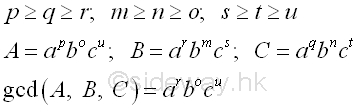Therefore every common factor of the set of numbers is a divisor of the greatest common divisor, gcd, of the set of numebrs.

#### Euclidean algorithm

Euclidean algorithm or Euclid's algorithm is an effective technique to determine the greatest common factor GCD of two numbers through iteration. The Euclidean algorithm makes use of the greatest common factor proporty of two numbers by assuming the two numbers as the multiples of the two numbers and then reducing the multiple factors through iteration. Imply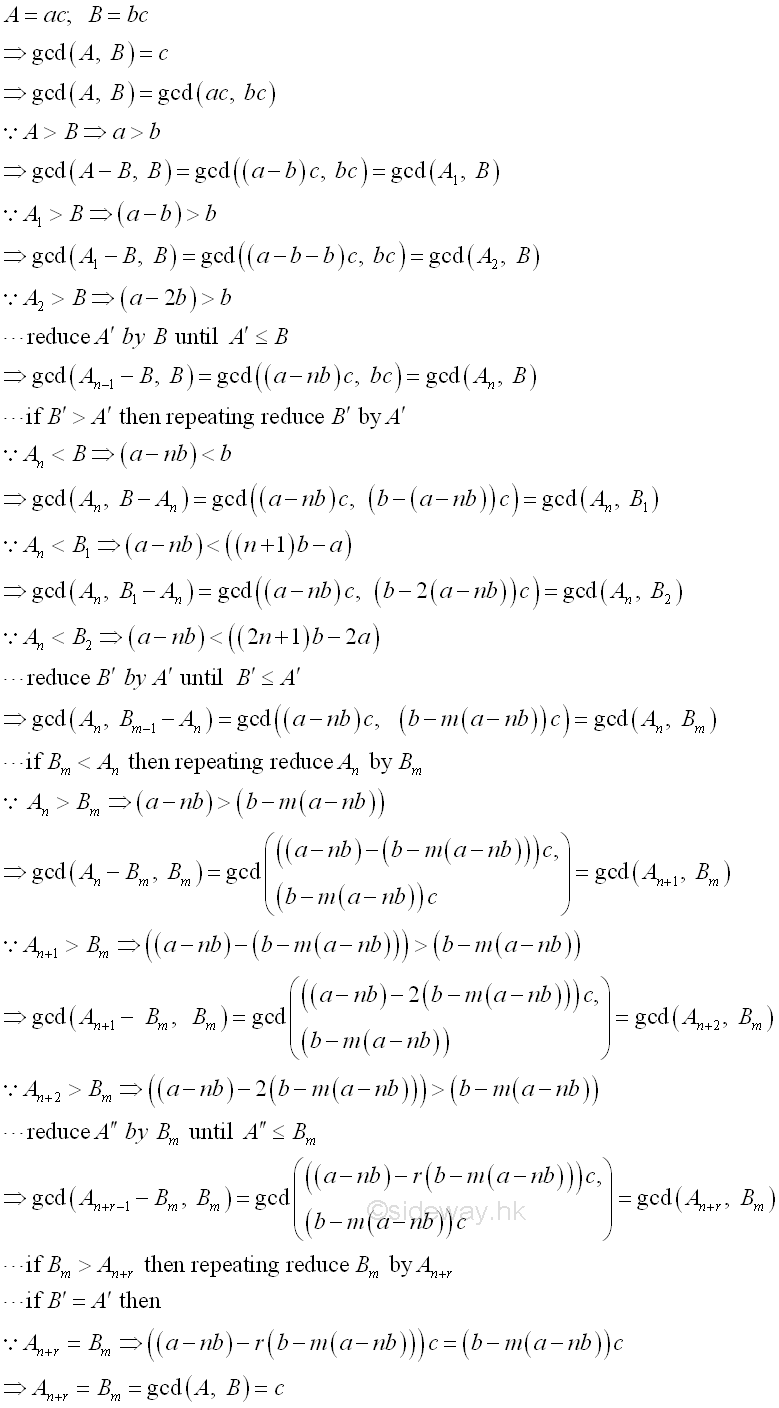The iterative steps of Euclidean algorithm or Euclid's algorithm can be expressed as a recursive equation, imply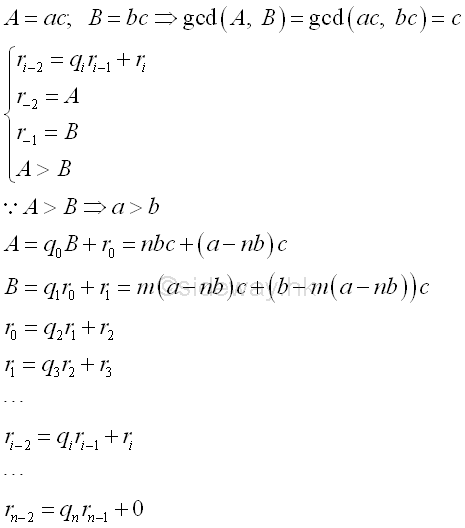#### Bezout's Identity

By reversing the Euclidean algorithm or Euclid's algorithm, the greatest common factor GCD of two numbers can be expressed the sume of two numbers which are obtained by multiplying a positive and a negative integers to the original numbers respectively in the form of ax+by=d where x and y are integers of Bezout coefficients of integers a and b and integer d is the common divisor of integers a and b. Imply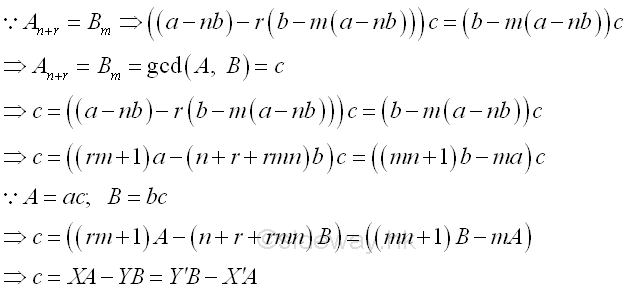## Least Common Multiple (LCM)

The least common multiple (lcm), or lowest common multiple (lcm), or smallest common multiple (scm) of a set of two or more non-zero integers is the least positive integer that can be divided by the set of integers without a remainder. In order to find the common multiple, the prime factorization should be used for determining the factors of all numbers. The least common multiple of the set of numbers can be obtained by multiplying the least common multiples of all prime factors of the set of numbers, i.e. multiply the highest power of common prime factors. imply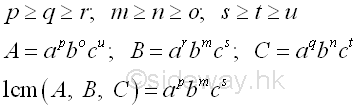If one of the number is equal to 0, then the least common multiple, lcm, is defined as zero.

Besides, the product of two numbers can be expressed the product of least common multiple and greatest common divisior of the two numbers. Imply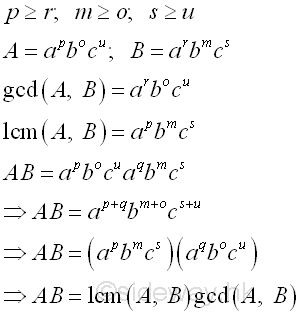## Modular Arithmetic

Modular arithmetic is also call clock arithmetic because modular arithmetic is a arithmetic for integers with number line wrap around like a clock, where number counting from 0 to a number m-1 for modulus m cyclically . e.g. number line of modulus 12 is 0, 1, 2, 3, 4, 5, 6, 7, 8, 9, 10, 11, 0, 1, 2, 3, 4, 5, 6, 7, 8, 9, 10, 11, 0, 1, 2,...

Let q be the quotient and r be the remainde when a number x is divided by modulus m. Imply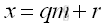The remainder can be represented by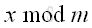Let p be the quotient and r be the remainder when a number y is divided by modulus m. Imply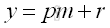The remainder can be represented by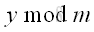For m≥2, both x and y have the same remainder when divided by m, the relationship between x and y can be expressed as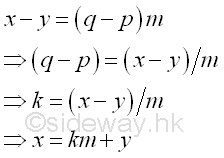Since q, and p are integers, k is integer also. In other words, m divides (x-y)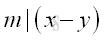Therefore both x and y are equivalent modulo m, x and y are said to be congruent modulo m, or x is congruent to y modulo m. Imply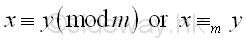©sideway

ID: 120400009 Last Updated: 2012/4/26 Revision:Home (5)

Business

Management

HBR (3)

Information

Recreation

Hobbies (7)

Culture

Chinese (1097)

English (336)

Reference (66)

Computer

Hardware (149)

Software

Application (187)

Digitization (24)

Numeric (19)

Programming

Web (644)CSS (SC)

ASP.NET (SC)

HTML

Knowledge Base

Common Color (SC)

Html 401 Special (SC)

OS (389)MS Windows

Windows10 (SC)

.NET Framework (SC)

DeskTop (7)

Knowledge

Mathematics

Formulas (8)

Number Theory (206)

Algebra (20)

Trigonometry (18)

Geometry (18)

Calculus (67)

Complex Analysis (21)

Engineering

Tables (8)

Mechanical

Mechanics (1)

Rigid Bodies

Statics (92)

Dynamics (37)

Fluid (5)

Control

Acoustics (19)

Biology (1)

Geography (1)

Latest Updated Links

Copyright © 2000-2019 Sideway . All rights reserved Disclaimers last modified on 10 Feb 2019